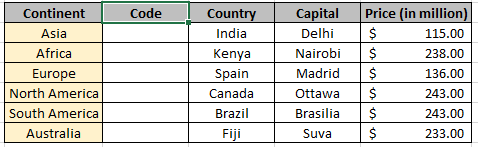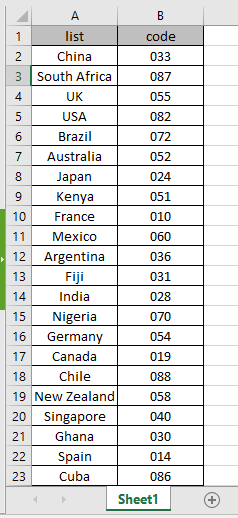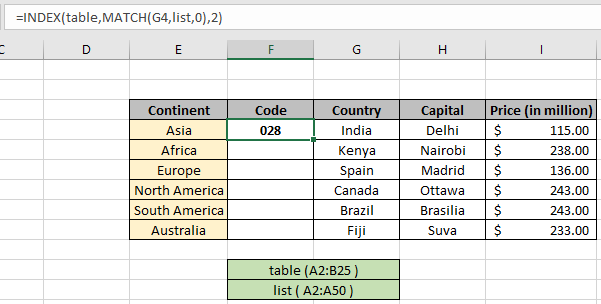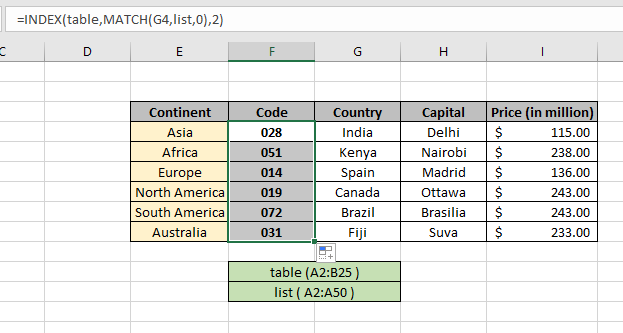# Auto populate table from another table using INDEX & MATCH function

In this article, we will learn how to Auto populate table from another table using the INDEX & MATCH function in Excel.
Scenario:

For instance, We need to find the EXACT match from the table without looking it up on the table. We need some fixed formula which helps in finding the exact match as to auto complete table.
How to Solve the Problem?

For the formula to understand first we need to revise a little about the following functions

Now we will make a formula using the above functions. MATCH function will return the index of the lookup value in the row header field. The INDEX function returns the value corresponding to will now be fed into the INDEX function to get the values under the lookup value from the table data.

Generic Formula:

= INDEX ( data , MATCH ( lookup_value, lookup_array, 0 ) , col_num ) )

data : array of values inside the table without headers

lookup_value : value to look for in look_array

look_array : array to look into

match_type : 1 ( exact or next smallest ) or 0 ( exact match) or -1 ( exact or next largest )

col_num : column number, required value to retrieve from the table column.

Example:

The above statements can be complicated to understand. So let’s understand this by using the formula in an example

Here we have a table having details about the world continent. We need to find the country code from the given table from the lookup value as country name.

Incomplete tableLookup_tableHere we used the named range instead for cell reference array as it's easy to understand. We are looking up for the value as shown in the snap below.

Use the above formula and named range to get the quantity of the order matching all criteria
Use the formula in the G6 cell:

= INDEX ( table , MATCH ( G4 , list , 0 ) , 2 )

Named ranges

table (A2:B25)

List (A2:A25)

Explanation:

• MATCH function matches the country name India in the list named range and returns its row index to the INDEX function.
• The INDEX function finds the value having ROW index and column number in the table named range
• The formula returns the value from the look_table.The formula returns Country code for the table to complete the table. Now copy the formula using the Ctrl + D or drag down the cell option in excel.As you can see from the above snapshot we obtained all the code details in the table. Auto populate table from another table using the above stated formula.

Here are some observational noter while using the above formula.

Notes:

1. The function returns the #NA error if the lookup array argument to the MATCH function is not of same length of the table array.
2. The formula returns an error if lookup_value doesn't match the value in the table lookup_array.
3. The function matches exact value as the match type argument to the MATCH function is 0.
4. The lookup values can be given as cell reference or directly using quote symbol ( " ) in the formula as arguments.

Hope you understood how to Auto populate table from another table using INDEX & MATCH function in excel. Explore more articles on Excel lookup value here. Please feel free to state your queries below in the comment box. We will certainly help you.

Related Articles

Use INDEX and MATCH to Lookup Value : INDEX & MATCH function to look up value as required.

SUM range with INDEX in Excel : Use INDEX function to find the SUM of the values as required.

How to use the INDEX function in Excel : Find the INDEX of array using the INDEX function explained with example.

How to use the MATCH function in Excel : Find the MATCH in the array using the INDEX value inside MATCH function explained with example.

How to use LOOKUP function in Excel : Find the lookup value in the array using the LOOKUP function explained with example.

How to use the VLOOKUP function in Excel : Find the lookup value in the array using the VLOOKUP function explained with example.

Popular Articles

50 Excel Shortcut to Increase Your Productivity

Edit a dropdown list

Absolute reference in Excel

If with conditional formatting

If with wildcards

Vlookup by date

Join first and last name in excel

Terms and Conditions of use

The applications/code on this site are distributed as is and without warranties or liability. In no event shall the owner of the copyrights, or the authors of the applications/code be liable for any loss of profit, any problems or any damage resulting from the use or evaluation of the applications/code.